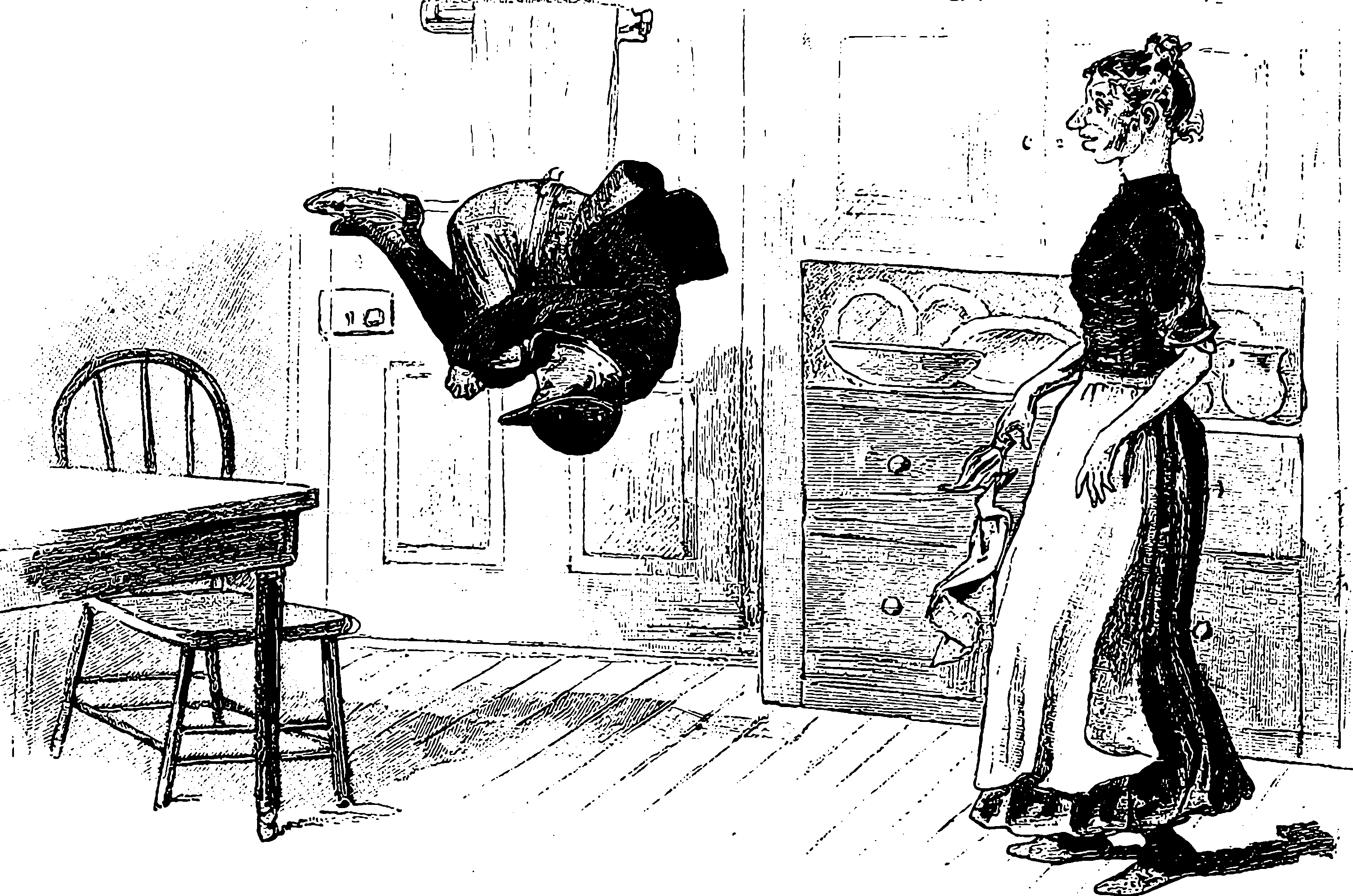# Random rotationsPlaceholder for random measures on the special orthogonal group, which encodes random $$d$$-dimensional rotations. A special type of matrix random variate if you’d like.

## Uniform random rotations

Key word: Haar measure.

Chatterjee and Meckes (2008) gives us a useful lemma describing the moments of a random rotation:

If $$U=\left[u_{i j}\right]_{i, j=1}^{n}$$ is an orthogonal matrix distributed according to Haar measure, then $$\mathbb{E}\left[\prod u_{i j}^{k_{i j}}\right]$$ is non-zero if and only if the number of entries from each row and from each column is even. Second and fourth-degree moments are as follows:

1. For all $$i, j$$, $\mathbb{E}\left[u_{i j}^{2}\right]=\frac{1}{n}$
2. For all $$i, j, r, s, \alpha, \beta, \lambda, \mu$$, \begin{aligned} &\mathbb{E}\left[u_{i j} u_{r s} u_{\alpha \beta} u_{\lambda \mu}\right]\\ &=- \frac{1}{(n-1) n(n+2)}\left[\delta_{i r} \delta_{\alpha \lambda} \delta_{j \beta} \delta_{s \mu}+\delta_{i r} \delta_{\alpha \lambda} \delta_{j \mu} \delta_{s \beta}+\delta_{i \alpha} \delta_{r \lambda} \delta_{j s} \delta_{\beta \mu}\right.\\ &\quad\left.+\delta_{i \alpha} \delta_{r \lambda} \delta_{j \mu} \delta_{\beta s}+\delta_{i \lambda} \delta_{r \alpha} \delta_{j s} \delta_{\beta \mu}+\delta_{i \lambda} \delta_{r \alpha} \delta_{j \beta} \delta_{s \mu}\right] \\ &+\frac{n+1}{(n-1) n(n+2)}\left[\delta_{i r} \delta_{\alpha \lambda} \delta_{j s} \delta_{\beta \mu}+\delta_{i \alpha} \delta_{r \lambda} \delta_{j \beta} \delta_{s \mu}+\delta_{i \lambda} \delta_{r \alpha} \delta_{j \mu} \delta_{s \beta}\right] \end{aligned}

## Tiny random rotations

Notation: $$\oplus$$ is the block direct sum.

### Random Givens rotation

Givens rotation are useful. We can parameterize a Givens rotation $$\mathrm{A}^{(t)}$$ by either an angle $$t=\theta$$ or a magnitude $$t=\epsilon=\sin \theta$$. The latter is slightly easier. Formally, a Givens rotation can be defined in any dimension $$d\geq 2$$ by padding it with zeros and adding it to an identity matrix, but all the action happens over 4 entries, so we can learn how it works by examining a minimal $$2\times2$$ rotation as a perturbation of the identity.

For $$-1\leq t\leq 1$$ fixed, let us define a rotation \begin{aligned} \mathrm{A}^{(t)} &=\left[\begin{array}{cc} \sqrt{1-t^{2}} & t \\ -t & \sqrt{1-t^{2}} \end{array}\right]\\ &=\mathrm{I}_{2}+\left[\begin{array}{cc} -\frac{t^{2}}{2}+\delta & t \\ -t & -\frac{t^{2}}{2}+\delta \end{array}\right] \\ \end{aligned} where $$\delta$$ is a deterministic constant and $$\delta=O\left(t^{4}\right).$$

Informally (because I do not have space or will to track lots of boring remainder terms) we note that for some fixed $$x=[x_1\,x_2]^\top$$ we have \begin{aligned} \mathrm{A}^{(t)}xx^\top\mathrm{A}^{(t)}{}^\top &\approx\left( \begin{array}{cc} x_1^2+2 t x_1 x_2+t^2 \left(-x_1^2+x_2^2\right) & x_1 x_2-2 t^2 x_1 x_2+t \left(-x_1^2+x_2^2\right) \\ x_1 x_2-2 t^2 x_1 x_2+t\left(-x_1^2+x_2^2\right) & -2 t x_1 x_2+x_2^2+t^2 \left(x_1^2-x_2^2\right) \\ \end{array} \right)\\ &=xx^\top+\left( \begin{array}{cc} 2 x_1 x_2 & -x_1^2+x_2^2 \\ -x_1^2+x_2^2 & -2 x_1 x_2 \\ \end{array} \right)t+\left( \begin{array}{cc} -x_1^2+x_2^2 & -2 x_1 x_2 \\ -2 x_1 x_2 & x_1^2-x_2^2 \\ \end{array} \right)t^2 \end{aligned}

If we want this to work in a higher dimension, we pad it like this: \begin{aligned} \mathrm{A}^{(t)} &=\left[\begin{array}{cc} \sqrt{1-t^{2}} & t \\ -t & \sqrt{1-t^{2}} \end{array}\right] \oplus \mathrm{I}_{d-2} \\ &=\mathrm{I}_{d}+\left[\begin{array}{cc} -\frac{t^{2}}{2}+\delta & t \\ -t & -\frac{t^{2}}{2}+\delta \end{array}\right] \oplus 0_{d-2} \end{aligned}

Now, let us consider how to randomise these. If we fix two axes and a random $$t$$ we can construct a random Givens rotation. Suppose $$\mathbb{E}[t]=0$$, $$\operatorname{var}(t)=\varepsilon^2$$ small, and proceeding to do a sloppy Taylor expansion (which will, I assert, not matter in practice when take limits as $$t\to 0$$ but one should check this to be rigorous). \begin{aligned} \mathbb{E}[\mathrm{A}^{(t)}x] &\approx\mathbb{E}\left[\left( \begin{array}{c} x_1+t x_2-\frac{t^2 x_1}{2} \\ x_2-t x_1-\frac{t^2 x_2}{2} \\ \end{array} \right)\right]\\ &=x-\mathbb{E}[t^2]x. \end{aligned}

\begin{aligned} \mathbb{E}[\mathrm{A}^{(t)}xx^\top\mathrm{A}^{(t)}{}^\top] &\approx \mathbb{E}\left[xx^\top+\left( \begin{array}{cc} 2 x_1 x_2 & -x_1^2+x_2^2 \\ -x_1^2+x_2^2 & -2 x_1 x_2 \\ \end{array} \right)t+\left( \begin{array}{cc} -x_1^2+x_2^2 & -2 x_1 x_2 \\ -2 x_1 x_2 & x_1^2-x_2^2 \\ \end{array} \right)t^2\right]\\ &= xx^\top + \left( \begin{array}{cc} -x_1^2+x_2^2 & -2 x_1 x_2 \\ -2 x_1 x_2 & x_1^2-x_2^2 \\ \end{array} \right)\mathbb{E}[t^2] \end{aligned}

### Doubly Random Givens rotation

If each Givens rotation is over a pair of distinct axes drawn uniformly at random, this will also give us a random rotation. Book keeping for these could be irritating, but let us see. wlog we can generate these by choosing the axes $$i\sim\mathcal{U}(\{1,2,\dots,n-1\},j\sim\mathcal{U}(\{i+1,i,\dots,n\}.$$

### Random rotation in an isotropic direction

Suppose $$\mathrm{Q}$$ is a Haar-distributed (i.e. uniform) random rotation. Then we can make a tiny incremental rotation using a construction of Chatterjee and Meckes (2008), by applying a small Given rotation in a randomly rotated coordinate frame. The result is a rotation in a random direction, but not far in that direction.

Then $$\mathrm{Q}^{\varepsilon}:=\mathrm{Q} \mathrm{A}_{\varepsilon} \mathrm{Q}^{\top}$$ is a small random rotation. What can we say about this guy? Let $$Q_{1:2}$$ be the $$d \times 2$$ matrix made of the first two columns of $$\mathrm{Q}$$ and $$C_{2}=\left[\begin{array}{cc}0 & 1 \\ -1 & 0\end{array}\right]$$. Then $\mathrm{Q}^{\varepsilon}=\epsilon\left[-\left(\frac{\epsilon}{2}+\epsilon^{-1} \delta\right) Q_{1:2} Q_{1:2}^{\top}+ Q_{1:2}C_2 Q_{1:2}^{\top}\right]$ and $$\epsilon^{-1} \delta=O\left(\epsilon^{3}\right)$$.

Once again referring to Chatterjee and Meckes (2008) we see that the matrix $$M=\left[m_{i j}\right]_{i, j=1}^{n}=M_{1:2}C_2 M_{1:2}^{\top}$$ satisfies $$m_{i j}=u_{i 1} u_{j 2}-u_{i 2} u_{j 1} .$$ For all $$i, j, \ell, p$$, $\mathbb{E}\left[m_{i j} m_{\ell p}\right]=\frac{2}{n(n-1)}\left[\delta_{i \ell} \delta_{j p}-\delta_{i p} \delta_{j \ell}\right]$ By the lemma on expectations of random rotations, $$\mathbb{E}\left[Q_{1:2} Q_{1:2}^{\top}\right]=\frac{2}{n} I$$.

TBC.

### No comments yet. Why not leave one?

GitHub-flavored Markdown & a sane subset of HTML is supported.